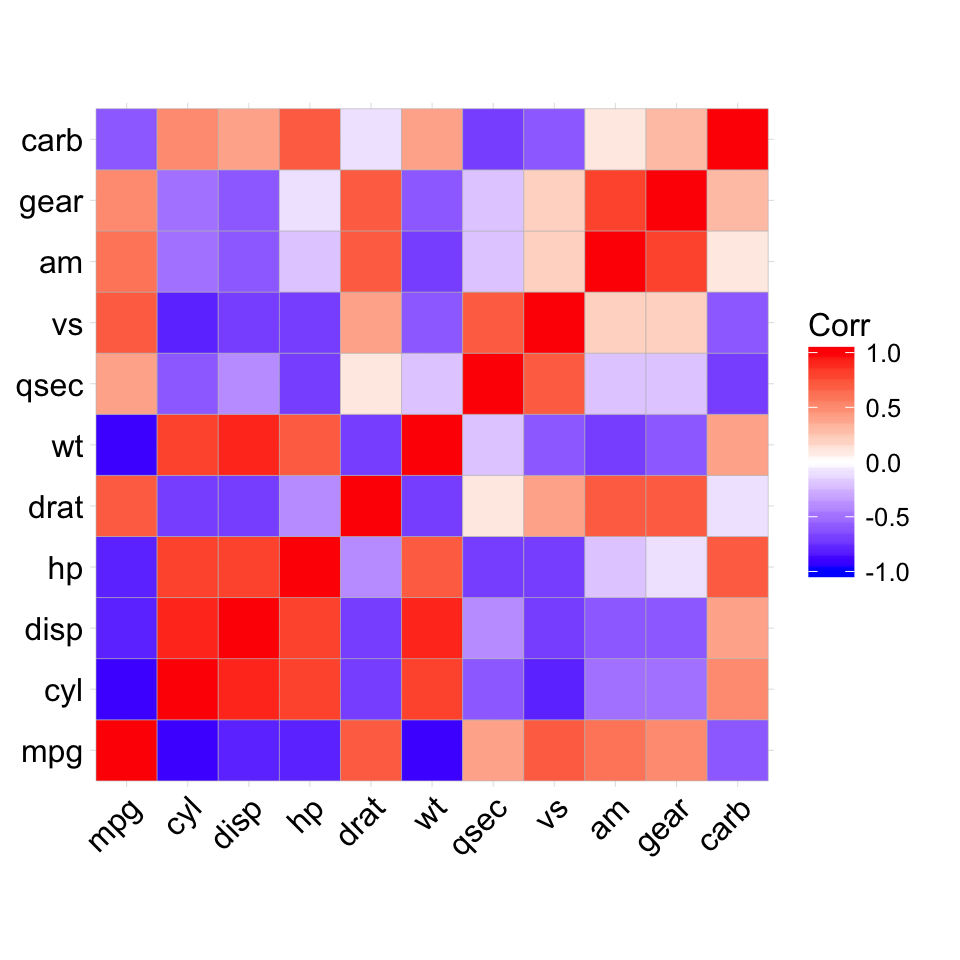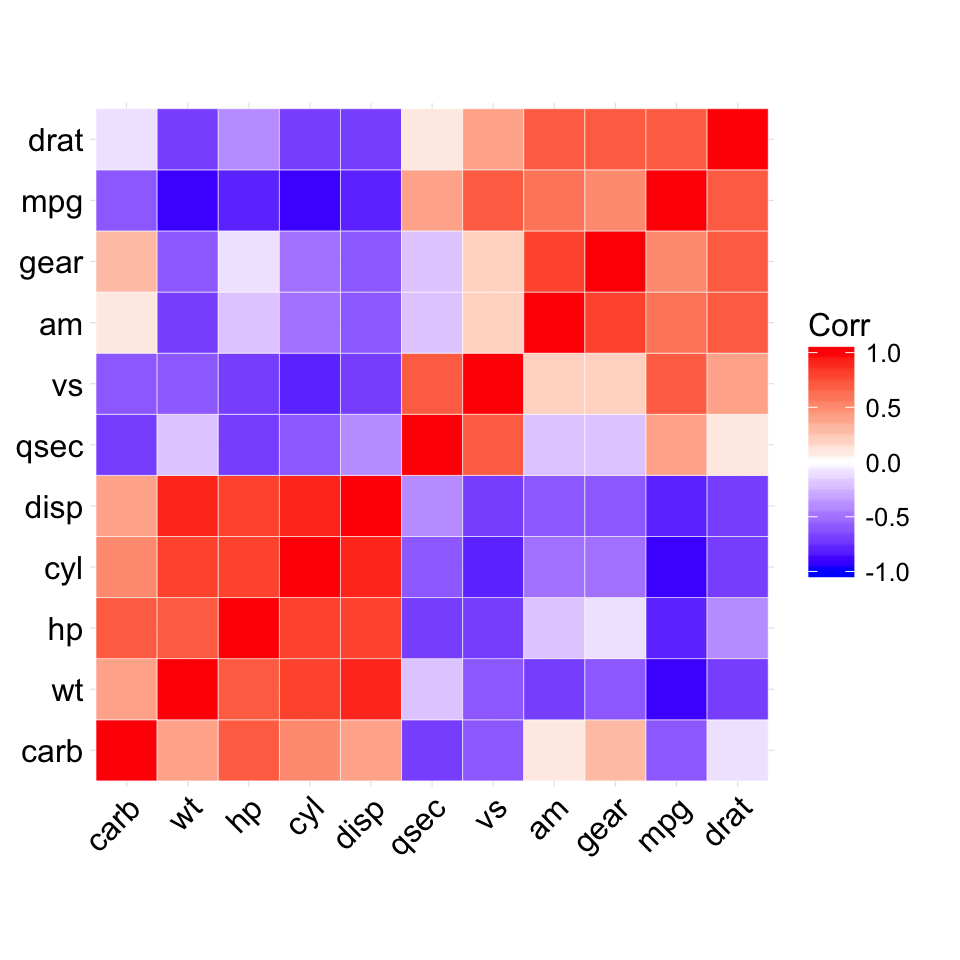# ggcorrplot: Visualization of a correlation matrix using ggplot2

The easiest way to visualize a correlation matrix in R is to use the package corrplot.

In our previous article we also provided a quick-start guide for visualizing a correlation matrix using ggplot2.

Another solution is to use the function ggcorr() in ggally package. However, the ggally package doesn’t provide any option for reordering the correlation matrix or for displaying the significance level.

In this article, we’ll describe the R package ggcorrplot for displaying easily a correlation matrix using ‘ggplot2’.

# ggcorrplot main features

It provides a solution for reordering the correlation matrix and displays the significance level on the correlogram. It includes also a function for computing a matrix of correlation p-values. It’s inspired from the package corrplot.

ggcorrplot can be installed from CRAN as follow:

``install.packages("ggcorrplot")``

``````# Install
if(!require(devtools)) install.packages("devtools")
devtools::install_github("kassambara/ggcorrplot")``````

``library(ggcorrplot)``

# Getting started

## Compute a correlation matrix

The mtcars data set will be used in the following R code. The function cor_pmat() [in ggcorrplot] computes a matrix of correlation p-values.

``````# Compute a correlation matrix
data(mtcars)
corr <- round(cor(mtcars), 1)
``````##       mpg  cyl disp   hp drat   wt
## mpg   1.0 -0.9 -0.8 -0.8  0.7 -0.9
## cyl  -0.9  1.0  0.9  0.8 -0.7  0.8
## disp -0.8  0.9  1.0  0.8 -0.7  0.9
## hp   -0.8  0.8  0.8  1.0 -0.4  0.7
## drat  0.7 -0.7 -0.7 -0.4  1.0 -0.7
## wt   -0.9  0.8  0.9  0.7 -0.7  1.0``````
``````# Compute a matrix of correlation p-values
p.mat <- cor_pmat(mtcars)
``````##               mpg          cyl         disp           hp
## mpg  0.000000e+00 6.112687e-10 9.380327e-10 1.787835e-07
## cyl  6.112687e-10 0.000000e+00 1.803002e-12 3.477861e-09
## disp 9.380327e-10 1.803002e-12 0.000000e+00 7.142679e-08
## hp   1.787835e-07 3.477861e-09 7.142679e-08 0.000000e+00
## drat 1.776240e-05 8.244636e-06 5.282022e-06 9.988772e-03
## wt   1.293959e-10 1.217567e-07 1.222311e-11 4.145827e-05``````

## Correlation matrix visualization

``````# Visualize the correlation matrix
# --------------------------------
# method = "square" (default)
ggcorrplot(corr)````````````# method = "circle"
ggcorrplot(corr, method = "circle")````````````# Reordering the correlation matrix
# --------------------------------
# using hierarchical clustering
ggcorrplot(corr, hc.order = TRUE, outline.col = "white")````````````# Types of correlogram layout
# --------------------------------
# Get the lower triangle
ggcorrplot(corr, hc.order = TRUE, type = "lower",
outline.col = "white")````````````# Get the upeper triangle
ggcorrplot(corr, hc.order = TRUE, type = "upper",
outline.col = "white")````````````# Change colors and theme
# --------------------------------
# Argument colors
ggcorrplot(corr, hc.order = TRUE, type = "lower",
outline.col = "white",
ggtheme = ggplot2::theme_gray,
colors = c("#6D9EC1", "white", "#E46726"))````````````# Add correlation coefficients
# --------------------------------
# argument lab = TRUE
ggcorrplot(corr, hc.order = TRUE, type = "lower",
lab = TRUE)````````````# Add correlation significance level
# --------------------------------
# Argument p.mat
# Barring the no significant coefficient
ggcorrplot(corr, hc.order = TRUE,
type = "lower", p.mat = p.mat)````````````# Leave blank on no significant coefficient
ggcorrplot(corr, p.mat = p.mat, hc.order = TRUE,
type = "lower", insig = "blank")``````